# The Assignment Operator and Statement The Most Common

• Slides: 18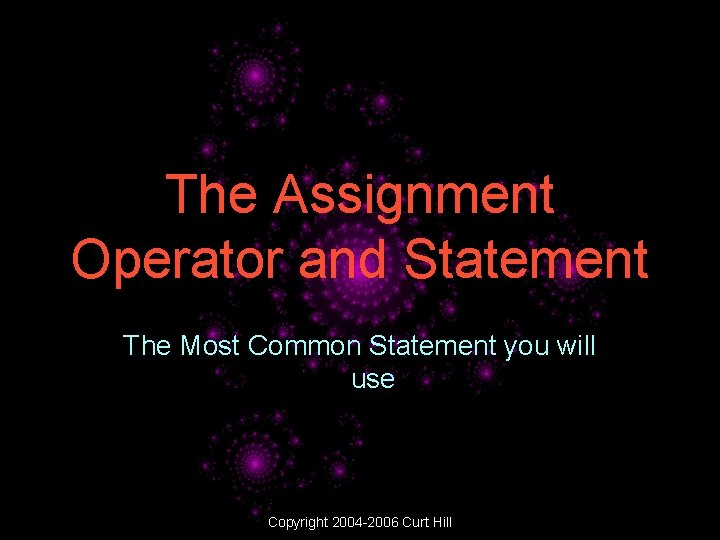The Assignment Operator and Statement The Most Common Statement you will use Copyright 2004 -2006 Curt Hill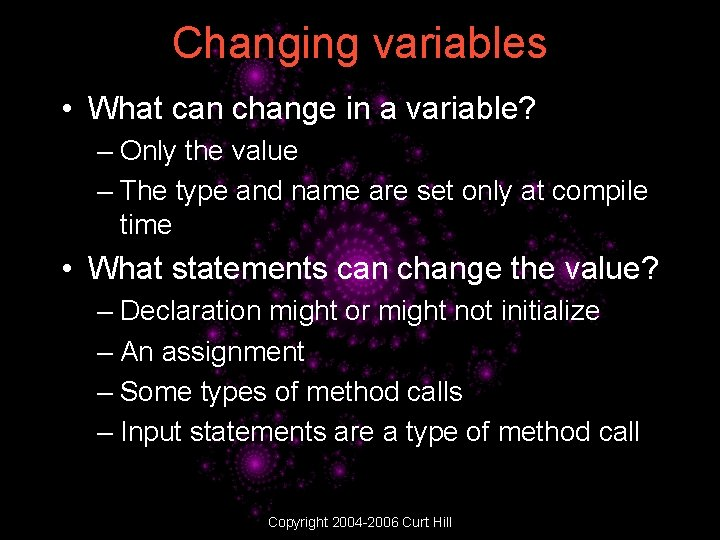Changing variables • What can change in a variable? – Only the value – The type and name are set only at compile time • What statements can change the value? – Declaration might or might not initialize – An assignment – Some types of method calls – Input statements are a type of method call Copyright 2004 -2006 Curt Hill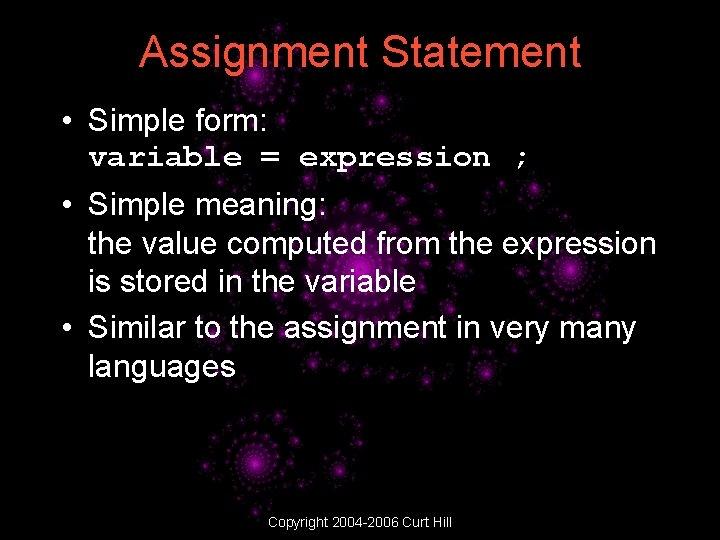Assignment Statement • Simple form: variable = expression ; • Simple meaning: the value computed from the expression is stored in the variable • Similar to the assignment in very many languages Copyright 2004 -2006 Curt HillWhat is an expression? • • • A legal combination of the following: Constants Variables Operators The legal combinations are usually intuitive Copyright 2004 -2006 Curt Hill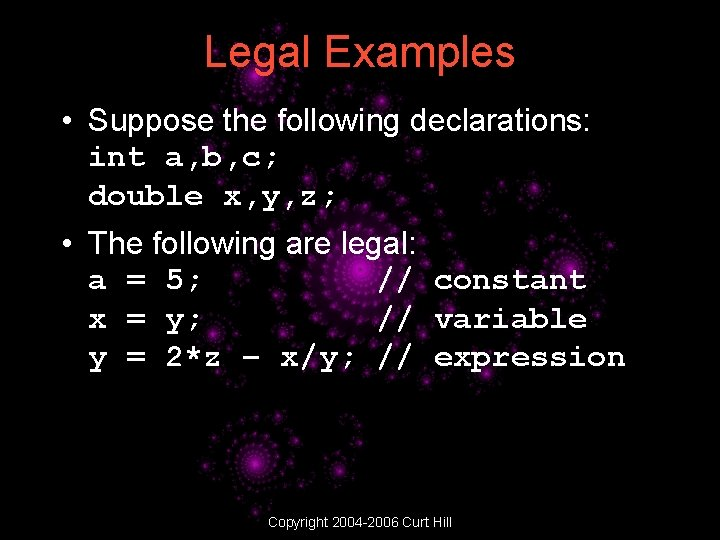Legal Examples • Suppose the following declarations: int a, b, c; double x, y, z; • The following are legal: a = 5; // constant x = y; // variable y = 2*z – x/y; // expression Copyright 2004 -2006 Curt Hill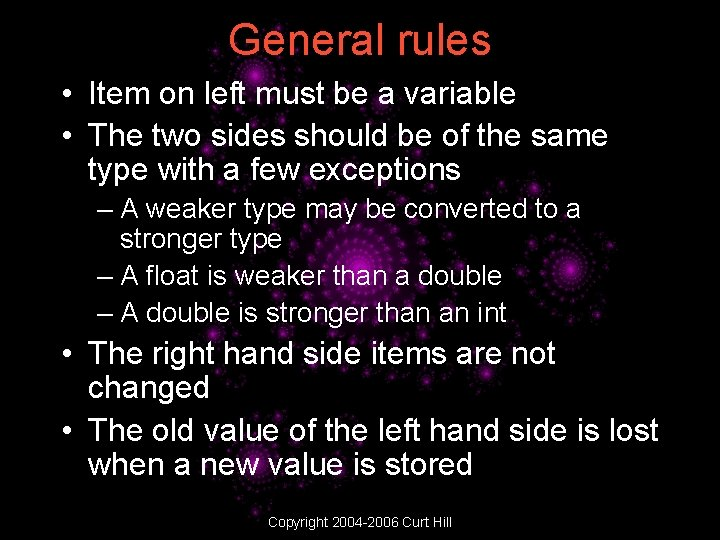General rules • Item on left must be a variable • The two sides should be of the same type with a few exceptions – A weaker type may be converted to a stronger type – A float is weaker than a double – A double is stronger than an int • The right hand side items are not changed • The old value of the left hand side is lost when a new value is stored Copyright 2004 -2006 Curt HillMore Examples • Suppose the following declarations: int a, b, c; double x, y, z; • The following are not legal: 5 = -c; // cannot change x = y // no semicolon x = y + * z; // malformed expr • The following are legal but with issues: x = a; // Stronger type a = 5. 8; // loses precision x = false; // incompatible Copyright 2004 -2006 Curt Hill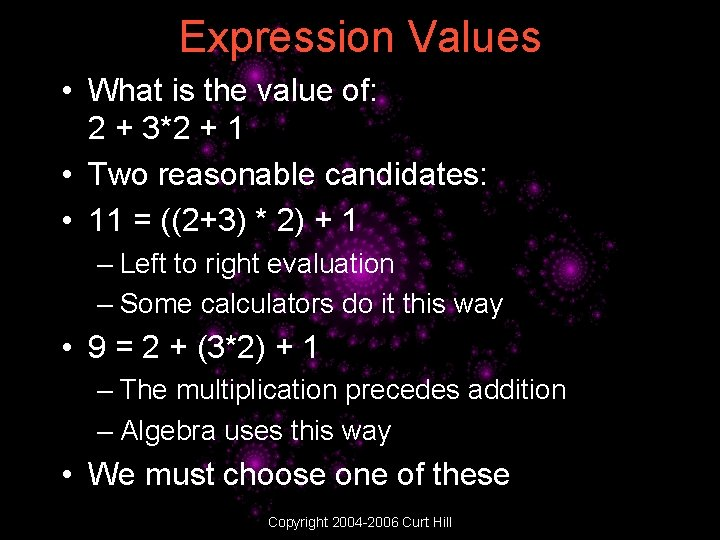Expression Values • What is the value of: 2 + 3*2 + 1 • Two reasonable candidates: • 11 = ((2+3) * 2) + 1 – Left to right evaluation – Some calculators do it this way • 9 = 2 + (3*2) + 1 – The multiplication precedes addition – Algebra uses this way • We must choose one of these Copyright 2004 -2006 Curt HillPrecedence • Clearly we cannot have both 11 and 9 correct or we never know what a computation produces • C++ like most (but not all) programming languages follows Algebra • Multiplication precedes addition • This gives rise to a precedence chart – Shows the level of importance for operators Copyright 2004 -2006 Curt Hill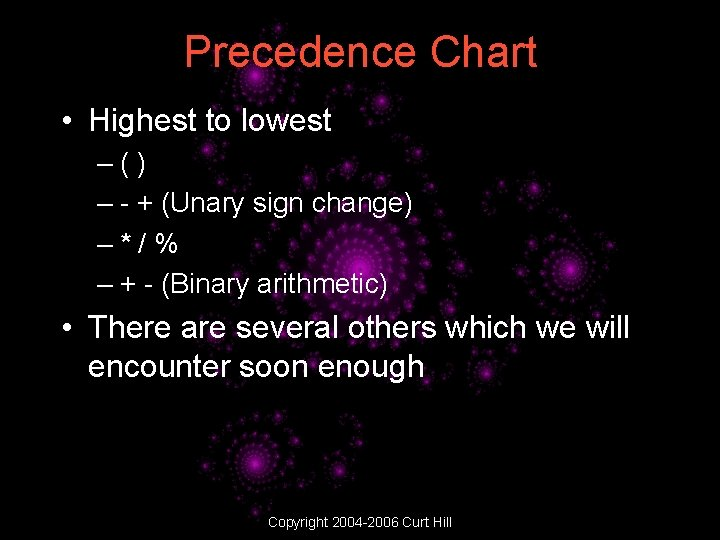Precedence Chart • Highest to lowest –() – - + (Unary sign change) –*/% – + - (Binary arithmetic) • There are several others which we will encounter soon enough Copyright 2004 -2006 Curt Hill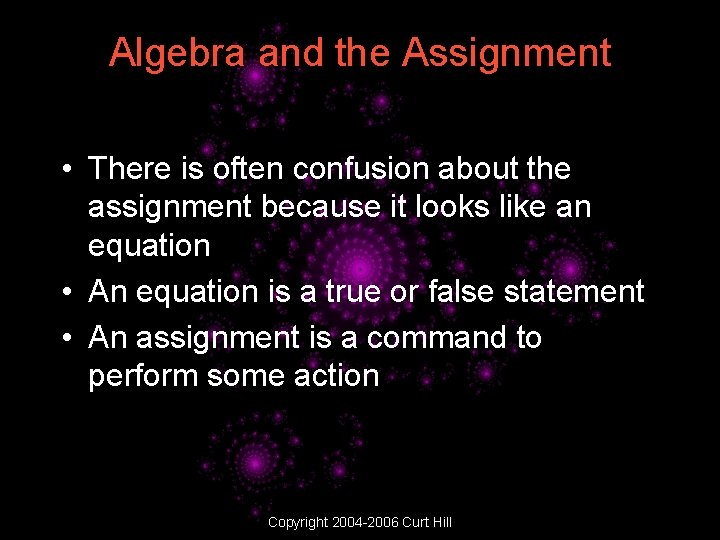Algebra and the Assignment • There is often confusion about the assignment because it looks like an equation • An equation is a true or false statement • An assignment is a command to perform some action Copyright 2004 -2006 Curt Hill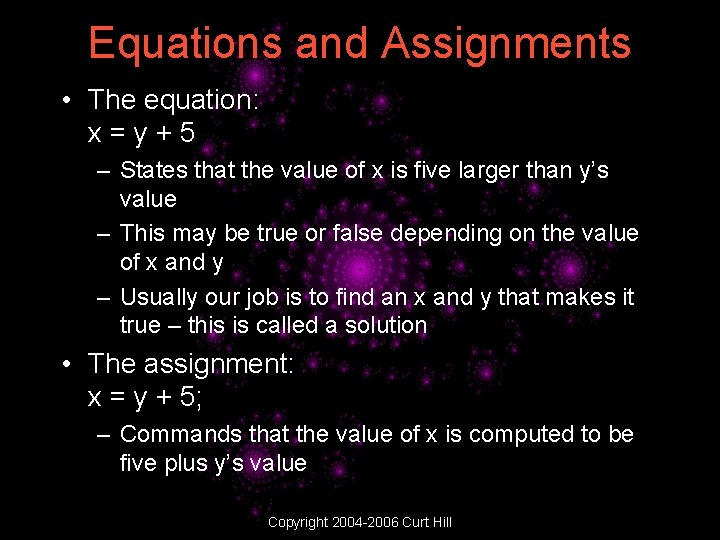Equations and Assignments • The equation: x=y+5 – States that the value of x is five larger than y’s value – This may be true or false depending on the value of x and y – Usually our job is to find an x and y that makes it true – this is called a solution • The assignment: x = y + 5; – Commands that the value of x is computed to be five plus y’s value Copyright 2004 -2006 Curt HillAnother Example • The equation: x=x+1 has no solution – It can never be true – No value may equal itself plus one • The assignment: x = x + 1; increments x – It is a command will be carried out Copyright 2004 -2006 Curt HillAnother Example • Suppose: int a=2, b=5, c=-7; • What happens to the variable values when: c = a*b - b%c+1; b = a-c/(1+a*2); is executed? • The value of c becomes 6 and the value of b becomes 1 Copyright 2004 -2006 Curt HillHow does this work? • int c = • b = • b = • b = a=2, b=5, c=-7; a*b - b%c+1; 2*5 – 5%-7+1 10 – 5 +1 6 a – c/(1+a*2); 2 – 6/(1+2*2) 2 – 6/(1+4) 2 – 6/5 2 – 1 1 Copyright 2004 -2006 Curt Hill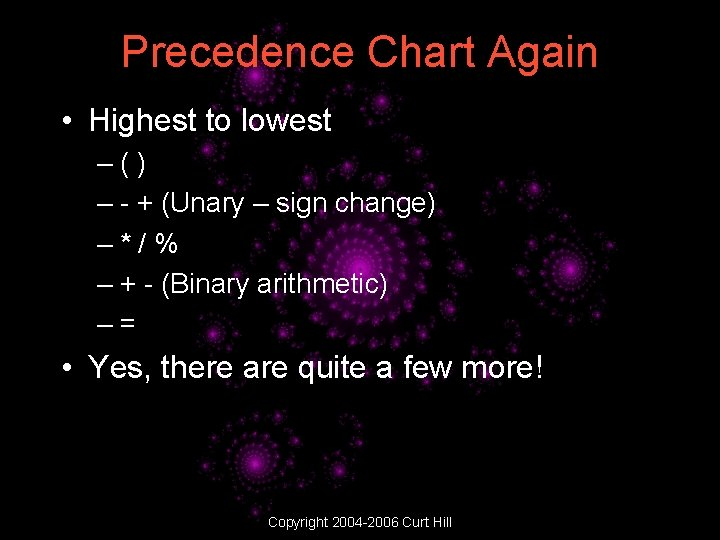Precedence Chart Again • Highest to lowest –() – - + (Unary – sign change) –*/% – + - (Binary arithmetic) –= • Yes, there are quite a few more! Copyright 2004 -2006 Curt Hill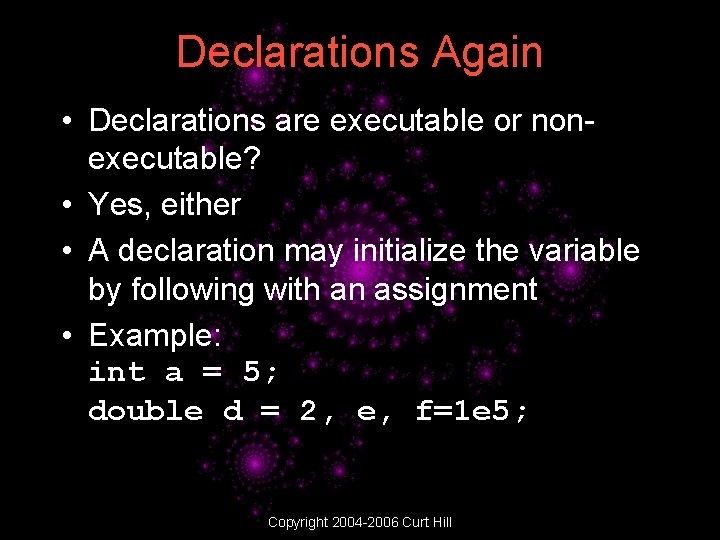Declarations Again • Declarations are executable or nonexecutable? • Yes, either • A declaration may initialize the variable by following with an assignment • Example: int a = 5; double d = 2, e, f=1 e 5; Copyright 2004 -2006 Curt Hill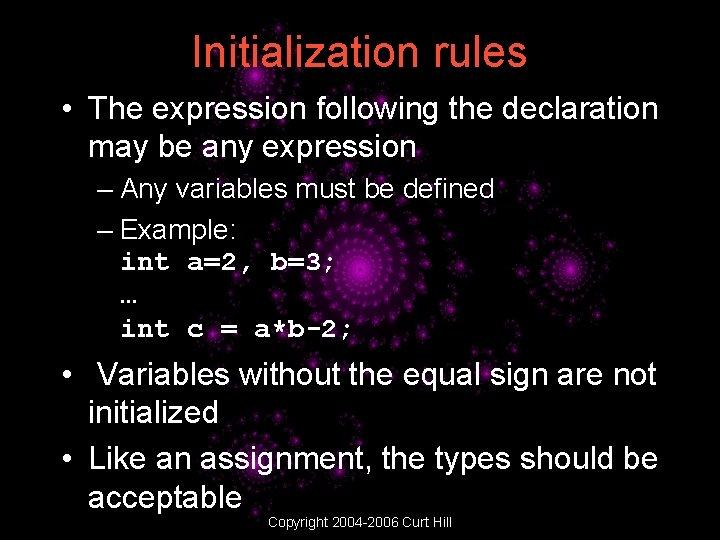Initialization rules • The expression following the declaration may be any expression – Any variables must be defined – Example: int a=2, b=3; … int c = a*b-2; • Variables without the equal sign are not initialized • Like an assignment, the types should be acceptable Copyright 2004 -2006 Curt Hill